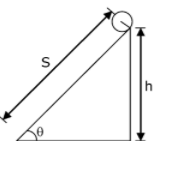Checkout JEE MAINS 2022 Question Paper Analysis : Checkout JEE MAINS 2022 Question Paper Analysis :

# The following bodies a. a ring b. a disc c. a solid cylinder d. a solid sphere of same mass 'm' and radius 'R' are allowed to roll down without slipping simultaneously from the top of the inclined plane. The body which will reach first at the bottom of the inclined plane is________.

[Mark the body as per their respective numbering given in the question]Acceleration of a body rolling down an inclined plane is,

$$\begin{array}{l}a = \frac{gsin\theta }{(1+\frac{I}{mR^{2}})}\end{array}$$

IR = mR2, aR = gsinθ/2

ID =mR2/2, aD = (⅔) g sin θ

ISC = mR2/2, asc = (⅔) g sin θ

ISS =(⅖)mR2, ass = (5/7) g sinθ

S = ut +(½) at2,

t = √2S/a

Therefore, t ∝ 1/√a For least time, a should be the highest

⇒ solid spheres will take minimum time.(1)(0)